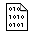## Firefly and PC GAMESS-related discussion club

Learn how to ask questions correctlyRe^2: Spin-orbit couplings only between states of consecutive CI calculations?

Bernhard Dick
bernhard.dick@chemie.uni-regensburg.de

It is a text-example: the lowest 2 states in each irreducible representation of singlet and triplet. For comparison I did it with Gamess-US 2018, and this worked. I include input and output of both.

with best regards,
Bernhard

On Thu Jul 26 '18 3:02pm, Alex Granovsky wrote
----------------------------------------------
>Dear Bernhard,

>could you please send me your input file (privately, if you like).

>Best regards,
>Alex
>
>
>
>On Wed Jul 25 '18 1:15am, Bernhard Dick wrote
>---------------------------------------------
>>Dear Alex,
>>I want to calculate the SOC between the singlet and triplet states of biphenylene. The molecule has D2 symmetry. I made orbitals by MCSCF with 12 electrons in 12 orbitals (i.e. full pi-space), then use these orbitals for the CI calculations.

>>When I switch off symmetry and do one calculation each for singlets and triplets, the CI "overlooks" the singlet state #4. Therefore, and for an easier symmetry assignment, I used one CI for each irreducible representation, i.e.

>> \$CIDRT1 GROUP=D2 ISTSYM=1 NMCC=35 NDOC=6 NALP=0 NVAL=6 FORS=.T. \$END
>> \$CIDRT2 GROUP=D2 ISTSYM=2 NMCC=35 NDOC=6 NALP=0 NVAL=6 FORS=.T. \$END
>> \$CIDRT3 GROUP=D2 ISTSYM=3 NMCC=35 NDOC=6 NALP=0 NVAL=6 FORS=.T. \$END
>> \$CIDRT4 GROUP=D2 ISTSYM=4 NMCC=35 NDOC=6 NALP=0 NVAL=6 FORS=.T. \$END
>> \$CIDRT5 GROUP=D2 ISTSYM=1 NMCC=35 NDOC=5 NALP=2 NVAL=5 FORS=.T. \$END
>> \$CIDRT6 GROUP=D2 ISTSYM=2 NMCC=35 NDOC=5 NALP=2 NVAL=5 FORS=.T. \$END
>> \$CIDRT7 GROUP=D2 ISTSYM=3 NMCC=35 NDOC=5 NALP=2 NVAL=5 FORS=.T. \$END
>> \$CIDRT8 GROUP=D2 ISTSYM=4 NMCC=35 NDOC=5 NALP=2 NVAL=5 FORS=.T. \$END
>> \$GUGDIA ITERMX=2000 cvgtol=1d-7 \$END
>> \$transt numvec=1 numci=8 nfzc=35 nocc=47 iroots(1)=2,2,2,2,2,2,2,2
>> Ā CASTRF=.T. \$end

>>However, Firefly now calculated coupling coefficient only within one CI, and with the following CI. E.g., within the CI#1 (singlet A-states) and between CI#1 (singlet-A) and CI#2 (singlet B1), but not between CI#1 and CI#6 (triplet B1 states) etc.

>>So what am I doing wrong?
>>with best regards,
>>Bernhard

>>This message contains the 121 kb attachment [ Biphenyl_D2_CI1212_SO.zip ]Fri Jul 27 '18 4:41pmThis message read 338 times